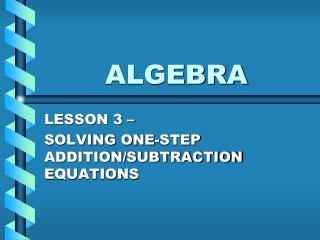DownloadDownload PresentationALGEBRA

# ALGEBRA

Télécharger la présentation## ALGEBRA

- - - - - - - - - - - - - - - - - - - - - - - - - - - E N D - - - - - - - - - - - - - - - - - - - - - - - - - - -
##### Presentation Transcript

1. ALGEBRA LESSON 3 – SOLVING ONE-STEP ADDITION/SUBTRACTION EQUATIONS

2. ONE-STEP EQUATIONS • An equation is a statement that says two expressions are equal • To solve an equation containing a variable means to find the value(s) of the variable that make(s) the equation true. EXAMPLE: x = 4 is a solution to the equation x + 6 = 10 because we all know that 4 + 6 = 10 x = 11 is not a solution because 11 + 6 is not equal to 10

3. SOLVING ONE-STEP EQUATIONS • A one-step equation is an equation that requires only one step to solve it

4. SOLVING EQUATIONS USING INVERSE OPERATIONS • In order to solve any equation, we must use inverse operations • the inverse of addition is subtraction • the inverse of subtraction is addition Essentially, you are trying to make it so the “x” is isolated. So, you are trying to make everything that is not attached to the “x” go away (turn into zero)

5. SOLVING EQUATIONS USING INVERSE OPERATIONS • If you see addition in an equation, you will use subtraction to solve it. • Same goes for subtraction, but you use addition. • REMEMBER: WHAT IS DONE TO ONE SIDE OF AN EQUATION MUST ALSO BE DONE TO THE OTHER SIDE • ALWAYS CHECK YOUR ANSWER TO MAKE SURE IT IS CORRECT

6. EXAMPLES OF ADDITION/SUBTRACTION EQUATIONS • x + 8 = 10 The 8 is not attached to the x. How can I turn 8 into a zero? Show this! What you do to one side, you must do to the other. x + 8 = 10 CHECK: - 8 -8 2 + 8 = 10? x = 2 10 = 10 yes

7. EXAMPLES OF ADDITION/SUBTRACTION EQUATIONS • x – 9 = 11 Since the 9 is not attached to the x, make it go away. What would make 9 (in this case – 9) into zero? Show this on both sides.

8. MORE EXAMPLES

9. ANY QUESTIONS?????? • HOMEWORK – Worksheet on Addition/Subtraction Equations